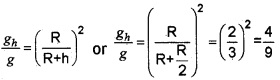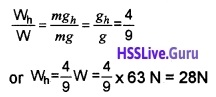# Plus One Physics Chapter Wise Questions and Answers Chapter 8 Gravitation

Students can Download Chapter 8 Gravitation Questions and Answers, Plus One Physics Chapter Wise Questions and Answers helps you to revise the complete Kerala State Syllabus and score more marks in your examinations.

## Kerala Plus One Physics Chapter Wise Questions and Answers Chapter 8 Gravitation

### Plus One Physics Gravitation One Mark Questions and Answers

Question 1.
If a satellite of mass m is revolving around the earth with distance rfrom centre, then total energy is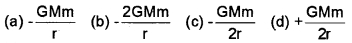(c) $$-\frac{G M m}{2 r}$$
The satellite revolving around the earth has two types of energies.
1. Gravitational potential energy U due to position of the satellite is given by
U = $$-\frac{\mathrm{GMm}}{\mathrm{r}}$$.

2. Kinetic energy K due to orbital velocity of satellite is given byQuestion 2.
The dimensions of universal gravitational constant are
(a) [M-2L3T-2]
(b) [M-2L2T-1]
(c) [M-1L3T-2]
(d) [ML2T-1]
(c) [M-1L3T-2]
According to Newton’s law of gravitation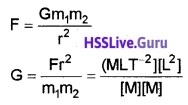Question 3.
When a radius of earth is reduced by 1% without changing the mass, then change in the acceleration due to gravity will be
(a) increased by 2%
(b) decreased by 1.5%
(c) increased by 1 %
(d) decreased by 1 %
(a) increased by 2%

Question 4.
The kinetic energy of a satellite is 2MJ. What is the total energy of the satellite?
(a) -2M J
(b) -1MJ
(c) -1/2MJ
(d) -4MJ
(a) -2MJ
Total energy of satellite – Kinetic energy of satellite.Question 5.
If total energy of this stone is negative, can it escape from the earth’s surface? Justify.
It will not escape as the force is attractive.

Question 6.
Why a man can jump higher on the moon than on earth?
The value of g on the moon is one sixth of that on earth.

Question 7.
The gravitational potential energy of a body of mass ‘m’ is -107J. What energy is required to project the body out of gravitational field of earth?
107J

Question 8.
How much energy is required by a satellite to keep it orbiting? Neglect air resistance.
No energy is required because work done by centripetal force is zero.

Question 9.
What would happen to an artificial satellites, if its orbital velocity is slightly decreased due to some defects in it?
It will fall on to earth.

Question 10.
Why a tennis ball bounces higher on hills than in plain?
As height increases ‘g’ decreases. The value of g on hills is less than that on the surface of earth. Hence tennis ball bounces higher on hills than in plains.Question 11.
The orbiting velocity of an earth-satellite is 8 km/s. What will be the escape velocity?
Ve = $$\sqrt{2}$$ V0
Ve = $$\sqrt{2}$$ × 8 = 11.3 kms-1.

### Plus One Physics Gravitation Two Mark Questions and Answers

Question 1.
1. A comet orbits the sun in a highly elliptical orbit. Does the comet have a constant

• linear speed
• total energy
• angular momentum

2. What are the consequences if the angular momentum is conserved?
1. Highly elliptical orbit:

• No
• Yes
• Yes

2. consequences if the angular momentum:

• Areal velocity of planet becomes constant
• Angular velocity changes according to the position of planet around sun.Question 2.
Fill in the blanks
R – Radius of earth, g – Acceleration due to gravity h – Height from surface of earth, d – Depth from surface of eartha) g(h) = g(1 – $$\frac{2 h}{R}$$)
b) Variation of g with depth
c) g Decreases with depth
d) (Change with radius (polar, equitorial org is maximum on the earth’s surface)

### Plus One Physics Gravitation Three Mark Questions and Answers

Question 1.
The Earth moving round the Sun in a circular orbit is acted upon by a force.

1. Name the force acting on the Earth.
2. Obtain a mathematical expression for the force.
3. What is the work done by the force?

1. Centripetal force
2. Fe = ma
Fc = m $$\frac{v^{2}}{r}$$
3. zero. (Displacement is always perpendicular to centripetal force).Question 2.
Escape velocity of objects in a planet depends on the mass and size of the planet.

1. Write down the expression for the escape velocity on the surface of the earth.
2. What is its value on the surface of our planet?
3. Give the reason why the moon does not have an atmosphere around it.

1. Escape velocity Ve = $$\sqrt{\frac{2 G M}{R}}$$
2. 11.2km/sec
3. The escape velocity of moon is very low. Hence all the gas molecules can escape from the moon’s surface.

Question 3.
The acceleration due to gravity is measured using ticker timer and is found to be 9.8ms-2

1. What does the value 9.8 for the acceleration implies?
2. One of your friends argues that the acceleration due to gravity at the centre of earth is infinity. Do you agree with it?

1. An acceleration of 9.8m2 implies that velocity change by 9.8ms1 in every second.

2. No, at centre g = 0

3. At centre d = R
gd = g × 0 = 0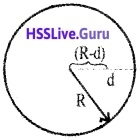### Plus One Physics Gravitation Four Mark Questions and Answers

Question 1.
A body of mass ‘m’ falls freely under gravity, near the surface of earth.

1. Will the acceleration of the body change if a part of the mass is thrown away from it?
2. What will be the free fall acceleration if it is falling from a height equal to R, the radius of earth?
3. If the mass is taken to the moon’s surface, will the free fall acceleration increase or decrease?

1. No. The acceleration of body does not depend on the mass of falling body.

2. We know acceleration due to gravity3. Decrease.Question 2.
Geo Stationary satellites are commonly used for communication purpose.

1. Name one geostationary satellite of earth.
2. What are the requirements of a geostationary satellite for its orbital motion.
3. Explain the phenomenon of ‘weightlessness’ in orbiting satellites.
4. Distinguish between gravitational mass and inertial mass.

1. INSAT.
2. The period of this satellite must be same as the period of the rotation of earth about its own axis. Its direction of rotation must be the same as that of the earth (from west to east).
3. The weight of satellite is used for providing centripetal force required for rotation. Hence anybody inside the satellite appears to be weightlessness.
4. The mass related with newtons second law of motion is called inertial mass. But the mass related with newtons law of gravitation is called gravitational mass.

Question 3.

1. What is acceleration due to gravity?
2. Does a body have the same weight at the equator and at the poles? Explain.
3. If the value of gravitational constant is 6.6 × 10-11 Nm2kg-2 and g = 9.8 m/s2, find the mass of the earth. Given, radius is 6.4 × 106m.

1. 9.8 m/se2

2. No. The weight of the body depends on the acceleration due to gravity at a place. The magnitude of acceleration due to gravity at equator and poles are different.

3.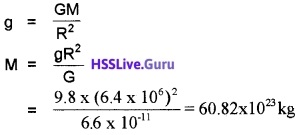Question 4.
Two identical satellites are orbiting in circular orbits around the earth at heights R and 3R respectively from the surface of the earth. The radius of the earth is R.

1. Define orbital velocity.
2. What is its value on the surface of the earth?
3. How do you compare the periods of revolution of these two satellites?

1. The minimum velocity required for a satellite to revolve around earth in a stable orbit is called orbital velocity.

2.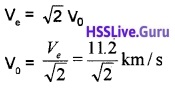3.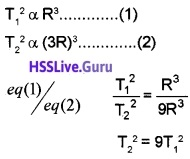Question 5.
The uniform acceleration produced in a freely falling body due to gravitational pull of the earth is known as acceleration due to gravity.

1. What is the value of acceleration due to gravity on the surface of earth.
2. Obtain an expression for acceleration due to gravity at a depth ‘d’ from the surface of earth
3. What is the value of gat the centre of earth?

1. 9.8m/s-2

2.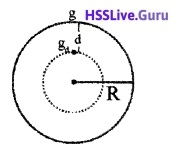If we assume the earth as a sphere of radius R with uniform density?
mass of earth = volume × density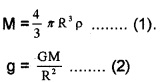Substituting eq(1) in eq(2), we get
g = $$\frac{4}{3}$$ πGRρ ……(3)
Therefore the acceleration due to gravity at a depth d is given by
gd = $$\frac{4}{3}$$ πG(R – d)ρ ……(4)
eq(4)/eq(3) and solving we get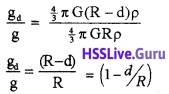gd = g(1 – d/R)
The above equation shows that, when depth increases g decreases.

3. Increase.

### Plus One Physics Gravitation Five Mark Questions and Answers

Question 1.
Earth can be treated as a sphere of radius R and mass M. A is a point at a height h above the Earth’s surface and B is another point at a depth h1 below the Earth’s surface. The acceleration due to gravity at Earth’s surface is g.

1. Obtain a formula to evaluate the acceleration due to gravity at A.
2. What is the value of acceleration due to gravity at B?
3. If we move from equator to pole, the value of g.
• increases
• decreases
• remains the same
• first increases and then decreases
• first decreases and then increases
4. What is the value of g at the centre of Earth? Explain.

1. The acceleration due to gravity on the surface of earth,
g = $$\frac{G m}{R^{2}}$$ …..(1)
At a height h, the acceleration due to gravity can be written as,eq(1)/eq(2) and solving we get
gh = g(1 – $$\frac{2 h}{R}$$)
This equation shows that acceleration due to gravity decreases as height increases. The above equation is valid when h << R.

2.3. Increase

4. Zero. At centre, the force acting on the body is zero.Question 2.
Fora particle to leave from earth’s gravitational field it should be projected with a minimum velocity.

1. Name the velocity.
2. Derive an expression for this velocity.
3. What is the magnitude of this velocity when this particle is projected from another planet whose mass and radius is twice that of the earth?

1. Escape velocity

2. Expression for escape speed:
Force on a mass m at a distance r from the centre of earth = $$\frac{G M m}{r^{2}}$$
Work done in taking the body to infinity from surface of earth,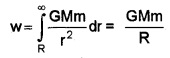This energy is given in the form of K.E. = $$\frac{1}{2}$$mve2,
where ve is the escape speed.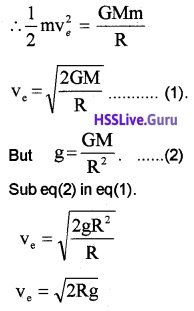This escape velocity $$\sqrt{2 \mathrm{Rg}}$$ is estimated to be 11.2 km/son the earth.

3. Escape velocity of planet,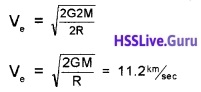Question 3.
Moon is the only satellite of earth. Mass of moon is very much lower than that of earth.

1. Is there any difference in gravitational force of moon and earth? Explain.
2. Deduce an equation for gravitational potential energy.
3. A girl argues that even today if we visit moon, we can see the foot steps of Neil Armstrong. What is your opinion?

1. Yes. Gravitational force of earth longer than gravitational force of moon.

2. Expression for gravitational P.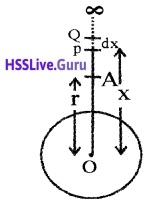Consider the earth as a uniform sphere of radius R and mass M. Considera point A at distance ‘r’ from the centre of earth. P is another point at a distance ‘x’ from O. Q lies at distance dx from P.

By definition, the gravitational potential energy of the body at point A, is the work done in bringing the body of mass ‘m’ from infinity to that point A The gravitational force on the body at the point P.
is given by F = $$\frac{G M m}{x^{2}}$$.
If the body is displaced from P to Q Work done, dw = F.dx
= $$\frac{G M m}{x^{2}}$$ Therefore, workdone in bringing the body from infinity to the point A,Since this workdone is stored inside the body as its gravitational potential energy, the gravitational potential energy (U) of a body of mass m at distance rfrom the centre of the earth is given by,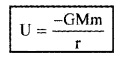The gravitational potential energy of a body at a point is defined as the amount of workdone in bringing the body from infinity to that point without acceleration.

3. There is no atmosphere on the moon. Hence we can see the foot steps of Neil Armstrong.

### Plus One Physics Gravitation NCERT Questions and Answers

Question 1.
Suppose there existed a planent that went around the Sun twice as fast as the earth. What would be its orbital size as compared to that of the earth?
Te = 1 year, Tp = $$\frac{1}{2}$$ year, Rp = ?, Re = 1 AU
Using Kepler’s third law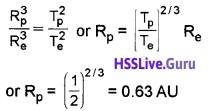Question 2.
lo, one of the satellites of Jupiter has an orbital period of 1.769 days and the radius of the orbit is 4.22 × 108m. Show that the mass of the Jupiter is about one-thousandth that of the Sun.Substituting the given data for Jupiter
Mass of JupiterSubstituting the known data forthe revolution of earth around Sun,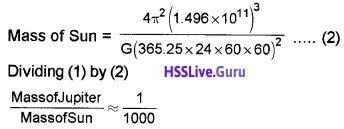Question 3.
Let us assume that our galaxy consists of 2.5 × 1011 stars each of one solar mass. How long will a star at a distance of 50,000 ly from the galactic centre take to complete on revolution? Take the diameter of the Milky way to be 105ly.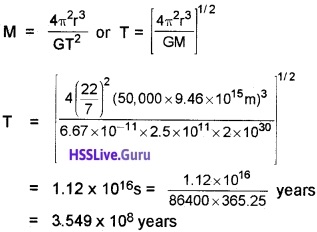Question 4.
A rocket is fired from the earth towards the Sun. At what distance from the earth’s centre is the gravitational force on the rocket zero? Mass of the Sun = 2 × 1030 kg, Mass of the earth = 6 × 1024kg. Neglect the effect of other planets etc. (Orbital radius = 1.5 × 1011m).
Mass of Sun, M = 2 × 1030 kg
Mass of Earth m = 6 × 1024kg.
Distance between Sun and Earth, r= 1.5 × 1011m
Suppose, at the point P, the gravitational force on the rocket due to earth = gravitational force on the rocket due to Sun
Let x be the distance of the point P from the earth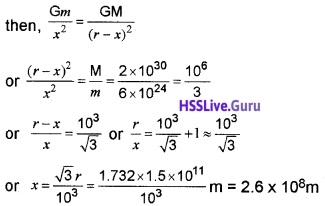Question 5.
How will you “weigh the Sun’, that is estimate its mass? The mean orbital radius of the earth around the Sun is 1.5 × 108km.
For the revolution of earth around sun, the gravitational force between the sun and the earth provides the necessary centripetal force.Question 6.
A geostationary satellite orbits the earth at a height of nearly 36,000km from the surface of the earth. What is the potentional due to earth’s gravity at the site of this sattelite? (Take the potential energy at infinity to be zero). Mass of the earth = 6 × 1024 kg., radius of earth = 6,400 km.
Required potential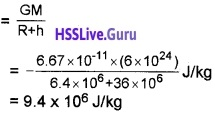Question 7.
A satum year is 29.5 times the earth year. How far is the saturn from the sun if the earth is 1.50 × 108km away from the sun?
We know that T2 α R3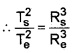where subscripts s and e refer to the satum and earth respectively.
Now $$\frac{T_{s}}{T_{e}}$$ = 29.5 (given); Re = 1.50 × 108km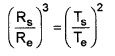Rs = Re × [(29.5)2]1/3 = 1.50 × 108 × (870.25)1/3km
= 1.43 × 109km = 1.43 × 1012m.Question 8.
A body weighs 63N on the surface of the earth. What is the gravitational force on it due to the earth at a height equal to half the radius of the earth?